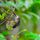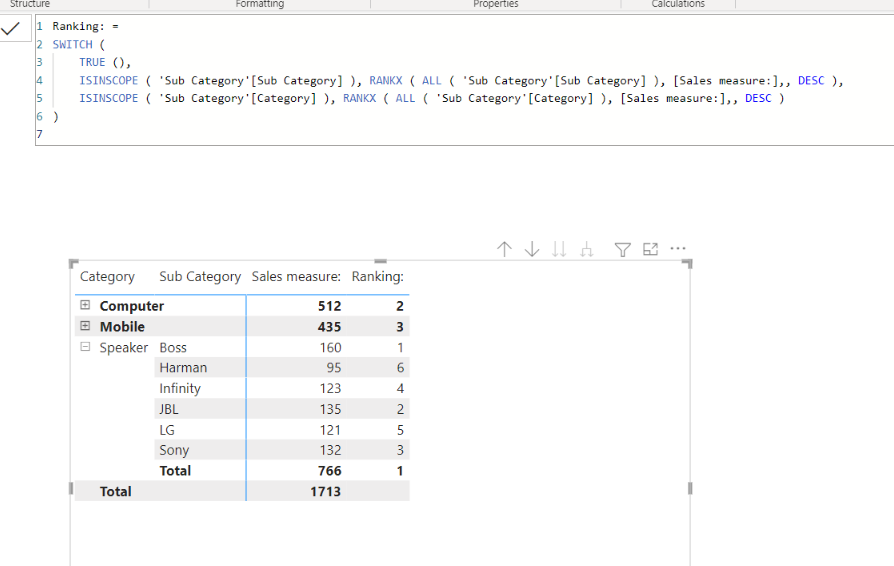cancel
Showing results for
Did you mean:New Member

## RANKX formula confusion

Dear Team,

I have a dataset in which Category, Sub Category and Sales are given.

I wish to create a matrix in which i want to see RANK based Category and Sub category when selected.

I have created one Fact table and 2 Dimention tables (Category table & Sub category table) and established relationships.

I want to see RANKS of each category in Matrix and while expanding the category button, rank should modify based on Sub category within same category.

Please note: sales column is from "Fact table", Sub category column from "Sub category table" and Category column from "Category table" as shown below.1 ACCEPTED SOLUTIONSuper User

Hi,

I suggest creating a data model like below.``````Sales measure: =
SUM( Data[Sales] )``````

``````Ranking: =
SWITCH (
TRUE (),
ISINSCOPE ( 'Sub Category'[Sub Category] ), RANKX ( ALL ( 'Sub Category'[Sub Category] ), [Sales measure:],, DESC ),
ISINSCOPE ( 'Sub Category'[Category] ), RANKX ( ALL ( 'Sub Category'[Category] ), [Sales measure:],, DESC )
)
``````

If this post helps, then please consider accepting it as the solution to help other members find it faster, and give a big thumbs up.

2 REPLIES 2Super User

Hi,

I suggest creating a data model like below.``````Sales measure: =
SUM( Data[Sales] )``````

``````Ranking: =
SWITCH (
TRUE (),
ISINSCOPE ( 'Sub Category'[Sub Category] ), RANKX ( ALL ( 'Sub Category'[Sub Category] ), [Sales measure:],, DESC ),
ISINSCOPE ( 'Sub Category'[Category] ), RANKX ( ALL ( 'Sub Category'[Category] ), [Sales measure:],, DESC )
)
``````

If this post helps, then please consider accepting it as the solution to help other members find it faster, and give a big thumbs up.New Member

Hi Jihwan,

thanks for your support on this. This gives a solution for my problem when the Category and Sub category columns are in same Dimention table. I actually wanted to do the same where Category and Sub category are two different Dimention tables.

But, since this gives a solution, i would like to go ahead with this.

thanks once again for your support.